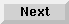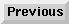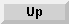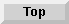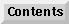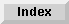5 Operators and Functions

## Defining a Function

When a numeric or string operation has to be evaluated several times, it is convenient to define it as a function. This is done using the DEF FN statement, which specifies a user-defined function and returns a single value as the value of the function. The simplest form is the single-line function, which can be used to define a numeric or string function. To define a numeric single-line function, use this syntax:

DEF FN function name [(formal parameter list)] = numeric expression

To define a string single-line function:

DEF FN function name\$ [(formal parameter list)] = string expression

The function name must be a valid name (as defined in page 73 ).

To use the defined function, use the FN statement.

Syntax of the FN statement for a numeric function is as follows:

FN function name [(pass parameter list)]

Syntax for the FN statement for a string function is as follows:

FN function name\$ [(pass parameter list)]

The values of the pass parameters are substituted for the formal parameters and the expression is evaluated. Its value is then returned as the value for the referencing syntax. page 177 provides a detailed explanation of parameters and also covers multi-line functions.

To show the use of single-line functions, consider the following program fragment:

```30   A=A+(SQR(A)-PI/20)
.
.
.
80   B=B+(SQR(B)-PI/20)
.
.
.
200   C=C+(SQR(C)-PI/20)
```
By defining:

```20   DEF FNNum(X)=X+(SQR(X)-PI/20)
function name  formal parameter
```
lines 30, 80, and 200 can be simplified:

```30   A=FNNum(A)
.           passed parameter
.
.
.
80   B=FNNum(B)
.           passed parameter
.
.
.
200   C=FNNum(C)
.            passed parameter
```
Recursion is not allowed in single line functions.

```300   DEF FNBad(X,Y,Z)=X+X+FNBad(A,B,C)*3
320   END
```
This would cause ERROR 48 IN LINE 310. FNBad must not reference itself either directly or indirectly via another function (in this case, FNBad calls FNWorse which then calls FNBad).

Single-line functions are local to the program segment in which they are defined (see section 7, Subprograms, on page 177 for an explanation of program segments.) For example:

```
10   DEF FNA(X)=PI*X
20   INPUT Z
30   Y=FNA(Z)
40   PRINT Y
50   CALL Set
60   END
70   SUB Set
80     INPUT I
90     J=FNA(I)
100    PRINT J
110   SUBEND
```
Lines 70 through 110 represent a subprogram.

When run, ERROR 7 IN LINE 90 (SUB Set) would occur since FNA is not defined in subprogram Set.

Multiple-line function subprograms can also be used to define a function. (see section , Multiple-Line Function Subprograms, on page 184)

Eloquence Language Manual - 19 DEC 2002